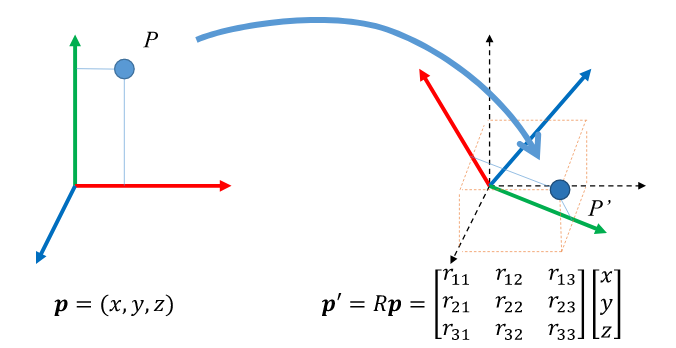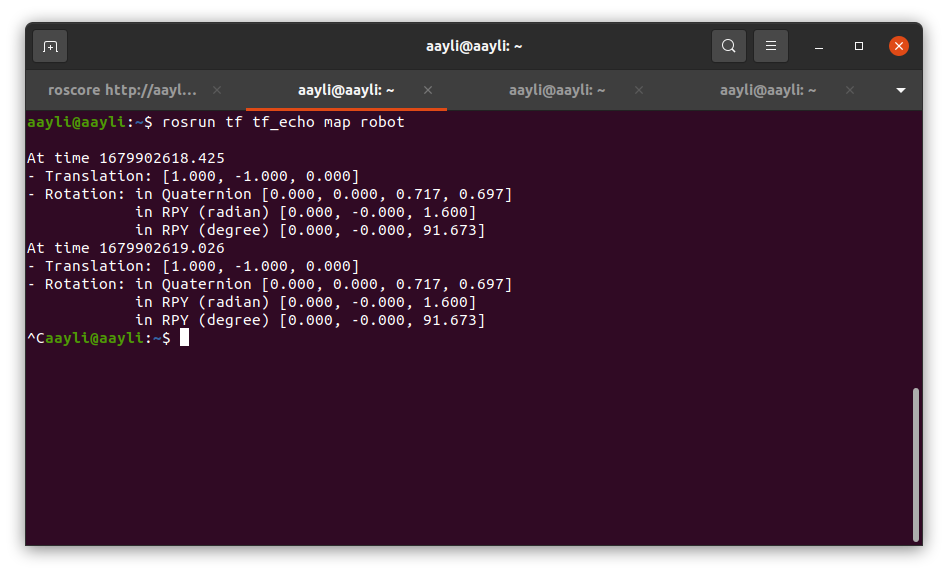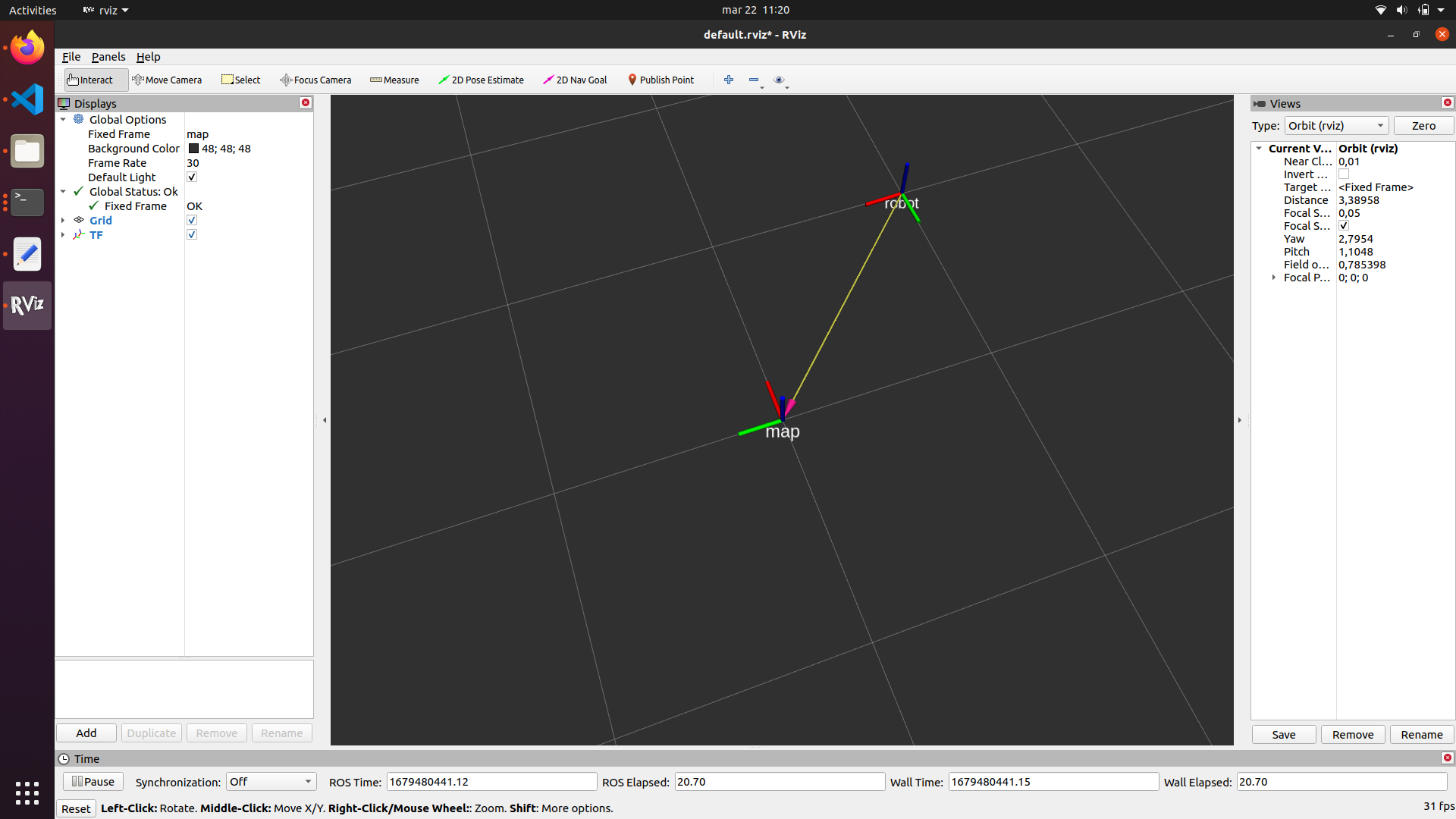# Transformation in ROS

## Chapter description​

This tutorial will cover the fairly simple concept of transformation. The selection of an appropriate reference point is crucial for many algorithms in the world of robotics. Therefore, it is very important that you have a good understanding of the current topic if you want to develop more advanced systems. Below, in addition to the theory, there are examples that implement simple frame transformations. This tutorial will be based on the tf package used in many ROS projects.

You can run this tutorial on:

Repository containing the final effect after doing all the ROS Tutorials you can find here

## Introduction​

Transformations are functions that map n-D vectors to other n-D vectors: T:Rn→Rn. They can represent geometric operations, which are caused by movement or action, as well as changes of coordinates, which are caused by changes of interpretation. Many common spatial transformations, including translations, rotations, and scaling are represented by matrix / vector operations. Changes of coordinate frames are also matrix / vector operations. As a result, transformation matrices are stored and operated on ubiquitously in robotics.

Coordinate transformation is used in many areas of mathematics, science, and engineering, and it has various applications. In this tutorial, transformations will be used to:

1. Changing perspective: Coordinate transformation can help us view the same object or phenomenon from different perspectives, which can be useful in many applications. For example, in physics, we might want to change the coordinate system to make the equations of motion simpler or to study a system in a different reference frame.
2. Mapping: Coordinate transformation can help map one coordinate system to another, which can be useful in navigation, cartography, and other fields.

A transformation is a simple operation consisting of a translation and a rotation. The picture below shows two frames of reference rotated relative to each other. To find the position of point P in the second frame of reference, multiply the point by the rotation matrix.The translation boils down to a simple addition of the coordinates of the second reference point.

In general, transformations involve simple matrix operations, and coordinate transformation itself is a powerful tool that can help us understand and work with complex systems and phenomena. Fortunately, the tf package has a lot of useful functions that do all these calculations for us.

## Basic transformation in ROS​

ROS can help track coordinate systems over time. The tf2 package is used for this - a library that use special geometry_msgs/TransformStamped message type. This message type consists:

• Header header - header message with timestamp for current transformation and information about parent frame id,
• string child_frame_id - the frame id of the child frame,
• Transform transform - transformation from coordinate frame header.frame_id to the coordinate frame child_frame_id.

### Static transformation​

This tutorial will show you how to add a static transformation between two frames. Suppose we have a robot that is fixed at (1,-1,0) relative to the map and rotated to the left. For this purpose, we can use the static_transform_publisher node from the tf package. Running the function is simple and just execute the command below:

rosrun tf static_transform_publisher x y z yaw pitch roll frame_id child_frame_id period_in_ms

or the same command but using quaternions:

rosrun tf static_transform_publisher x y z qx qy qz qw frame_id child_frame_id period_in_ms

Now all you have to do is complete the above fields in the appropriate way.

husarion@husarion:~$rosrun tf static_transform_publisher 1 -1 0 1.6 0 0 map robot 100 ### Visualization​ #### TF_Echo​ A simple tool that allows you to quickly check the transformation between any two frames. husarion@husarion:~$
rosrun tf tf_echo map robot#### TF_Tree​

The tool shows all the relationships between the individual forms in a diagram. This tool allows you to check if all frames are properly connected to each other.

husarion@husarion:~$rosrun rqt_tf_tree rqt_tf_tree#### RViz​ The RVIZ program known from chapter 4 will be used for visualization. To run RViz just type in new terminal. rviz Now click Add. In the new window, select TF from the list.Task 1 Try adding another reverse_camera frame which is 20 cm above the robot and facing the back of the robot. Use static_transform_publisher and check the result with all the tools presented above. Solution In new terminal type: rosrun tf static_transform_publisher 0 0 0.2 3.14 0 0 robot reverse_camera 100 Remember that if you point to a non-existing frame, a new relation will be created that is not related in any way to the currently existing transformations. To display it, change the Fixed Frame field in RViz ## Broadcasting transformation​ As you can see, the static transformation is very simple, but what to do when we want to simulate the mutual position of moving objects. ROS also allows this, but this time we need to create a node in which we will describe the movement of one frame relative to the other. Suppose we want to create an object that moves around the map. In this case, it is necessary to create a so-called TransformBroadcaster, which is a special sender type for sending tf messages. Despite the name difference, it works very similar to the classic Publisher works very similar to publisher except that it can only send tf messages. Let's start with creating a new file and name it tf_broadcaster.cpp. ~/ros_ws/src/tutorial_pkg/src/tf_broadcaster.cpp #include <ros/ros.h>#include <tf/transform_broadcaster.h>#include <math.h>void sendTransformation(){ static tf::TransformBroadcaster tf_broadcaster; tf::Transform transform; double t = ros::Time::now().toSec(); // Set translation transform.setOrigin(tf::Vector3(cos(t), sin(t), 0.0)); // Set rotation using roll, pitch. yaw tf::Quaternion q; q.setRPY(0, 0, fmod(t, 2*M_PI)+M_PI_2); transform.setRotation(q); tf_broadcaster.sendTransform(tf::StampedTransform(transform, ros::Time::now(), "map", "robot"));}int main(int argc, char **argv){ ros::init(argc, argv, "tf_broadcaster"); ros::NodeHandle n("~"); ros::Rate loop_rate(100); while (ros::ok()) { ros::spinOnce(); sendTransformation(); loop_rate.sleep(); }} The code explained static tf::TransformBroadcaster tf_broadcaster;tf::Transform transform; A unique publisher type and its message necessary to send transformation messages. double t = ros::Time::now().toSec(); Receiving a time in seconds. transform.setOrigin(tf::Vector3(cos(t), sin(t), 0.0)); Setting translation of frame to Transform message. Since we want the object to rotate around the center of the map, the variables at the center must be time dependent. tf::Quaternion q;q.setRPY(0, 0, fmod(t, 2*M_PI)+M_PI_2);  Creating quaternion message for storing rotation data and setting the deflection angle in such a way that the x-axis is in line with the direction of movement. transform.setRotation(q); Setting rotation to Transform message. tf_broadcaster.sendTransform(tf::StampedTransform(transform, ros::Time::now(), "map", "robot")); Publishing of transform is done with sendTransform method. We send TransformStamped, so instead of transform we need to pass time, child_frame and parent_frame. Build code Now in CMakeLists.txt file find line find_package(catkin REQUIRED COMPONENTS roscpp) and change it to find_package(catkin REQUIRED COMPONENTS roscpp tf) Declare executable and specify libraries to link. add_executable(tf_broadcaster src/tf_broadcaster.cpp)target_link_libraries(tf_broadcaster${catkin_LIBRARIES})

Run code and try adding another frame using static_transform_publisher and see the result in RViz.

Solution

You can use the same command as in the Task 1.

rosrun tf static_transform_publisher 0 0 0.2 3.14 0 0 robot reverse_camera 100

## Listening transformation​

As was the case with sending, receiving TF data is also a bit different from the Subscriber you already know. A TransformListener is used to receive data, which will automatically determine the transformations between two frames, even if they are not directly connected to each other.

~/ros_ws/src/tutorial_pkg/src/tf_listener.cpp
#include <ros/ros.h>#include <tf/transform_listener.h>void listenTransformation(tf::TransformListener& tf_listener){   // Listen transformation   tf::StampedTransform transform;   tf_listener.lookupTransform("map", "robot", ros::Time(0), transform);   // Get rotation: roll, pitch, yaw   tf::Quaternion q = transform.getRotation();   tf::Matrix3x3 m(q);   double roll, pitch, yaw;   m.getRPY(roll, pitch, yaw);   // Calcualte number of rotation   static int num_of_rotations;   static float prev_yaw;   if(yaw-prev_yaw < 0){      num_of_rotations++;      ROS_INFO("Number of rotations: %d", num_of_rotations);   }   prev_yaw = yaw;}int main(int argc, char **argv){   ros::init(argc, argv, "tf_listener");   ros::NodeHandle n("~");   ros::Rate loop_rate(100);      tf::TransformListener tf_listener;   tf_listener.waitForTransform("map", "robot", ros::Time(0), ros::Duration(2.0));   while (ros::ok())   {      ros::spinOnce();      listenTransformation(tf_listener);      loop_rate.sleep();   }}

The code explained

tf::TransformListener tf_listener;tf_listener.waitForTransform("map", "robot", ros::Time(0), ros::Duration(2.0));

Creat listener and wait for 2 secounds to find latest transformation between two frames. Function returns false if it failed to find a transformation.

tf::StampedTransform transform;tf_listener.lookupTransform("map", "robot", ros::Time(0), transform);

Create a variable and writing the last received transformation to it. The third argument means time at which we want to transform. Providing ros::Time(0) will just get us the latest available transform.

tf::Quaternion q = transform.getRotation();tf::Matrix3x3 m(q);double roll, pitch, yaw;m.getRPY(roll, pitch, yaw);

Reading quaternions and converting them to roll, pitch, yaw

Build code

Declare executable and specify libraries to link.

add_executable(tf_listener src/tf_listener.cpp)target_link_libraries(tf_listener    \${catkin_LIBRARIES})

Look at the values ​​of the yaw angle and change the code so that it determines the number of revolutions.

Solution

Repleace line ROS_INFO("Yaw: %f", yaw); with below code and build your file.

// Calcualte number of rotationstatic int num_of_revolutions;static float prev_yaw;if(yaw-prev_yaw < 0){    num_of_revolutions++;    ROS_INFO("Number of revolutions: %d", num_of_revolutions);}prev_yaw = yaw;

## Summary​

It's all for today. I hope that after this tutorial and the reworked exercises, handling and interpreting the tf package will not be a big problem for you. At this stage, you should perfectly understand the concept of transformation, what frames are, and you have the right tools for data visualization.

by Łukasz Mitka, Rafał Górecki, Husarion

Do you need any support with completing this tutorial or have any difficulties with software or hardware? Feel free to describe your thoughts on our community forum: https://community.husarion.com/ or to contact with our support: support@husarion.com